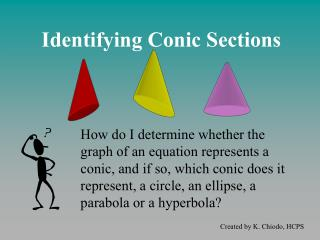DownloadDownload PresentationIdentifying Conic Sections

# Identifying Conic Sections

Download Presentation## Identifying Conic Sections

- - - - - - - - - - - - - - - - - - - - - - - - - - - E N D - - - - - - - - - - - - - - - - - - - - - - - - - - -
##### Presentation Transcript

1. Identifying Conic Sections How do I determine whether the graph of an equation represents a conic, and if so, which conic does it represent, a circle, an ellipse, a parabola or a hyperbola? Created by K. Chiodo, HCPS

2. We usually see conic equations written in General, or Implicit Form: General Form of a Conic Equation where A, B, C, D, E and F are integers and A, B and C are NOT ALL equal to zero. Note: You may see some conic equations solved for y, but if the equation can be re-written into the form above, it is a conic equation!

3. Please Note: A conic equation written in General Form doesn’t have to have all SIX terms! Several of the coefficients A, B, C, D, E and F can equal zero, as long as A, B and C don’t ALL equal zero. If A, B and C all equal zero, what kind of equation do you have? ... T H I N K... Linear!

4. So, it’s a conic equation if... • the highest degree (power) of x and/or y is 2 (at least ONE has to be squared) • the other terms are either linear, constant, or the product of x and y • there are no variable terms with rational exponents (i.e. no radical expressions) or terms with negative exponents (i.e. no rational expressions)

5. The values of the coefficients in the conic equation determine the TYPE of conic. What values form an Ellipse? What values form a Hyperbola? What values form a Parabola?

6. Ellipses... where A & C have the SAME SIGN NOTE: There is noBxy term, and D, E & Fmay equal zero! For example:

7. This is an ellipse since x & y are both squared, and both quadratic terms have the same sign! Center (-2, 0) Hor. Axis = 2 Vert. Axis = √8 The General, or Implicit, Form of the equations can be converted to Graphing Form by completing the square and dividing so that the constant = 1. Ellipses...

8. Vert. axis = 2/√3 center (-1, 1) Hor. axis = 2 In this example, x2 and y2 are both negative (still the same sign), you can see in the final step that when we divide by negative 4 all of the terms are positive. Ellipses...

9. Radius = √5 Center (-2, 0) Ellipses…a special case! When A & C are the samevalue as well as the same sign, the ellipse is the same length in all directions … Circle! it is a ...

10. Hyperbola... where A & C have DIFFERENT signs. NOTE: There is noBxy term, and D, E & Fmay equal zero! For example:

11. This is a hyperbola since x & y are both squared, and the quadratic terms have different signs! y-axis=3 x-axis=2 Center (2,-1) The General, or Implicit, Form of the equations can be converted to Graphing Form by completing the square and dividing so that the constant = 1. Hyperbola...

12. x-axis=2 Center (0,3) y-axis=2 In this example, the signs change, but since the quadratic terms still have different signs, it is still a hyperbola! Hyperbola...

13. A parabola is vertical if the equation has an x squared term AND a linear y term; it may or may not have a linear x term & constant: A parabola is horizontal if the equation has a y squared term AND a linear x term; it may or may not have a linear y term & constant: A Parabola can be oriented 2 different ways: Parabola...

14. Parabola …Vertical The following equations all represent vertical parabolas in general form; they all have a squared x term and a linear y term:

15. Vertex (2,3) Parabola …Vertical To write the equations in Graphing Form, complete the square for the x-terms. There are 2 popular conventions for writing parabolas in Graphing Form, both are given below:

16. Vertex (-1,4) Parabola …Vertical In this example, the signs must be changed at the end so that the y-term is positive, notice that the negative coefficient of the x squared term makes the parabola open downward.

17. Parabola …Horizontal The following equations all represent horizontal parabolas in general form, they all have a squared y term and a linear x term:

18. Vertex (1,-4) Parabola …Horizontal To write the equations in Graphing Form, complete the square for the y-terms. There are 2 popular conventions for writing parabolas in Graphing Form, both are given below:

19. Vertex (3,0) Parabola …Horizontal In this example, the signs must be changed at the end so that the x-term is positive; notice that the negative coefficient of the y squared term makes the parabola open to the left.

20. What About the term Bxy? None of the conic equations we have looked at so far included the term Bxy. This term leads to a hyperbolic graph: or, solved for y:

21. where A, B, C, D, E and F are integers and A, B and C are NOT ALL equal to zero. Summary ... General Form of a Conic Equation: Identifying a Conic Equation:

22. Practice ... Identify each of the following equations as a(n): (a) ellipse (b) circle (c) hyperbola (d) parabola (e) not a conic See if you can rewrite each equation into its Graphing Form!

23. Answers ... (a) ellipse (b) circle (c) hyperbola (d) parabola (e) not a conic

24. P H Conic Sections ! C E Created by K. Chiodo, HCPS